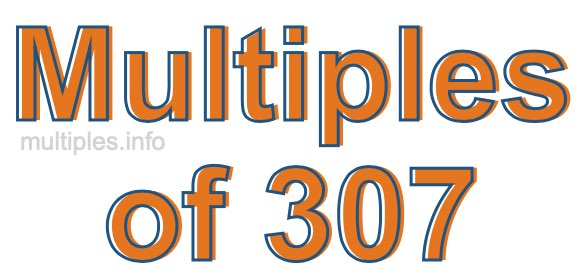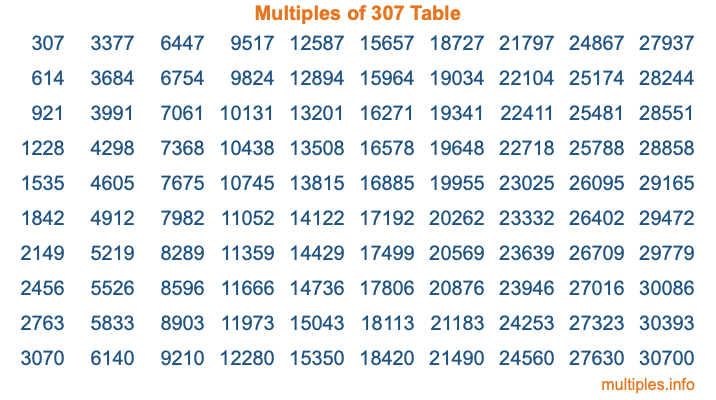Multiples of 307Welcome to the Multiples of 307 page. Here we will first teach you everything you will ever need to know about the multiples of 307, and then give you a study guide summary of everything we taught you to make sure you remember it all. Use this page to look up facts and learn information about the multiples of 307. This page will make you a multiples of three hundred seven expert!

Definition of Multiples of 307
Multiples of 307 are all the numbers that when divided by 307 equal an integer. Each of the multiples of 307 are called a multiple. A multiple of 307 is created by multiplying 307 by an integer.

Therefore, to create a list of multiples of 307, you start with 1 multiplied by 307, then 2 multiplied by 307, then 3 multiplied by 307, and so on for as long as you want. Thus, the list of the first five multiples of 307 is 307, 614, 921, 1228, and 1535. To see a larger list of multiples of 307, see the printable image of Multiples of 307 further down on this page. We also have a category where you can choose any nth multiple of 307.

Multiples of 307 Checker
The Multiples of 307 Checker below checks to see if any number of your choice is a multiple of 307. In other words, it checks to see if there is any number (integer) that when multiplied by 307 will equal your number. To do that, we divide your number by 307. If the the quotient is an integer, then your number is a multiple of 307.

Is  a multiple of 307?

Least Common Multiple of 307 and ...
A Least Common Multiple (LCM) is the lowest multiple that two or more numbers have in common. This is also called the smallest common multiple or lowest common multiple and is useful to know when you are adding our subtracting fractions. Enter one or more numbers below (307 is already entered) to find the LCM.

Check out our LCM Calculator if you need more details about the Least Common Multiple or if you need the LCM for different numbers for adding and subtraction fractions.

nth Multiple of 307
As we stated above, 307 is the first multiple of 307, 614 is the second multiple of 307, 921 is the third multiple of 307, and so on. Enter a number below to find the nth multiple of 307.

th multiple of 307

Multiples of 307 vs Factors of 307
307 is a multiple of 307 and a factor of 307, but that is where the similarities end. All postive multiples of 307 are 307 or greater than 307. All positive factors of 307 are 307 or less than 307.

Below is the beginning list of multiples of 307 and the factors of 307 so you can compare:

Multiples of 307: 307, 614, 921, 1228, 1535, etc.

Factors of 307: 1, 307

As you can see, the multiples of 307 are all the numbers that you can divide by 307 to get a whole number. The factors of 307, on the other hand, are all the whole numbers that you can multiply by another whole number to get 307.

It's also interesting to note that if a number (x) is a factor of 307, then 307 will also be a multiple of that number (x).

Multiples of 307 vs Divisors of 307
The divisors of 307 are all the integers that 307 can be divided by evenly. Below is a list of the divisors of 307.

Divisors of 307: 1, 307

The interesting thing to note here is that if you take any multiple of 307 and divide it by a divisor of 307, you will see that the quotient is an integer.

Multiples of 307 Table
Below is an image of the first 100 multiples of 307 in a table. The table is in chronological order, column by column. The first column has the first ten multiples of 307, the second column has the next ten multiples of 307, and so on.The Multiples of 307 Table is also referred to as the 307 Times Table or Times Table of 307. You are welcome to print out our table for your studies.

Negative Multiples of 307
Although not often discussed or needed in math, it is worth mentioning that you can make a list of negative multiples of 307 by multiplying 307 by -1, then by -2, then by -3, and so on, to get the following list of negative multiples of 307:

-307, -614, -921, -1228, -1535, etc.

Multiples of 307 Summary
Below is a summary of important Multiples of 307 facts that we have discussed on this page. To retain the knowledge on this page, we recommend that you read through the summary and explain to yourself or a study partner why they hold true.

There are an infinite number of multiples of 307.

A multiple of 307 divided by 307 will equal a whole number.

307 divided by a factor of 307 equals a divisor of 307.

The nth multiple of 307 is n times 307.

The largest factor of 307 is equal to the first positive multiple of 307.

307 is a multiple of every factor of 307.

307 is a multiple of 307.

A multiple of 307 divided by a divisor of 307 equals an integer.

307 divided by a divisor of 307 equals a factor of 307.

Any integer times 307 will equal a multiple of 307.

Multiples of a Number
Here you can get the multiples of another number, all with the same attention to detail as we did for multiples of 307 on this page.

Multiples of
Multiples of 308
Did you find our page about multiples of three hundred seven educational? Do you want more knowledge? Check out the multiples of the next number on our list!